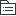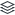# 小说

|
8

## 相关模板推荐

•## 小说 —— 作品大纲

•情节
• 情节梳理
• 思路
• 线索
• 场面
• 事件
• 情节作用
• 开头部分
• 统领全文，领起下文
• 奠定感情基调，渲染气氛
• 设置悬念，埋下伏笔。
• 中间部分
• ①承上启下，贯穿全篇
• ②详略得当，主次分明
• ③充实内容，寄托情感
• ④先抑后扬/先抑，对比映衬
• ⑤铺垫下文，行文线索
• 结尾部分
• 照应题目，呼应上文
• 深化主旨，卒章显志
• 情节叙述的角度
• 第一人称
• 便于抒情；也有利于隐藏作者的意图，留给读者去推测
• 第三人称
• 上帝视角，全知全能，寓情于事
• 情节叙述的方式
• 顺序
• 倒序
• 插叙
• 补叙
• 平叙
• 情节的设置技巧
• 一波三折式
• （全篇）引人入胜，增强戏剧性，使事件发展的方向改变
• 首尾呼应式
• （全篇）结构紧密，完整
• 倒序式
• （开头）造悬念，引起读者兴趣
• 抑扬式
• （全篇）造成落差，突出人物，增强文章波折美和吸引力
• 伏笔
• 照应
• 欧亨利
• 意料之外，情理之中，情节陡转突出人物，丰富内容
• 留白式
• 戛然而止，给读者想象空间，更好深化主题
•线索
• 存在形式
• 人物
• 事物
• 主要事件
• 时空
• 人物心理
• 作用
• 人物
• 突显人物形象
• 主题
• 巧妙安排结构，揭示主题
• 情节
• 使情节集中，避免赘述
• 读者
• 使小说结构清晰，便于读者阅读
• 暗线
• 在更广更深的层面上揭示当时社会各种斗争或矛盾的焦点
•标题
• 交代主要人物形象
• 概括小说主要事件
• 点明时间地点，创设故事背景，渲染氛围
• 贯穿全文，充当线索
• 象征意义
• 揭示主题，深化主题
• 寄托作者情感，奠定文章基调
• 展开情节，前后呼应
• 对比讽刺，强化效果
• 吸引读者
•人物
• 概括人物形象性格
• 正侧面结合
• 整体与细节结合
• 个性延伸至普遍性与典型性
• 形象的作用
• 思路
• 人物与人物
• 衬托……
• 人物与故事情节
• 推动故事情节发展
• 埋伏笔
• 制造悬念
• 人物与主题
• 对文章主题有表现作用
• 塑造形象的技巧
• 正面描写（正面着意）
• 细节描写（外动神语心）
• 侧面描写（侧面烘托）
• 景物烘托
• 人物烘托
• 正衬
• 反衬
• 比喻，对比，排比等修辞
• 形象探究
• 从人物分析
• 从情节分析
• 从环境分析
• 从主题分析
•环境
• 自然环境
• 烘托人物形象
• 渲染故事气氛
• 推动情节发展
• 暗示社会环境
• 深化主题
• 社会环境
• 交代人物生存环境
• 交代社会关系
• 交代作品时代背景
• 暗示人物行为动机
• 暗示人物或喜或悲的命运
•主题
• 人物
• 环境
• 情节
• 标题
• 作者倾向
•品味文中重要语句
• 内容上
• 情感可能复杂，矛盾，多变，表现了人物怎样的形象
• 主旨上
• 可能是中心句，主旨句
• 结构上
• 可能是总起句，过度句，总结句（提现文章脉络）
• 表达上
• 意味隽永，比喻句，象征句
•鉴赏小说语言特色
• 修辞
• 句式
• 整散句
• 长短句
• 语体色彩
• 口音
• 书面语
• 感官
• 听觉
• 嗅觉
• 视觉
• 触觉
• 心理感受
• 整体语言风格鉴赏
• 通俗，华丽，幽默，辛辣……